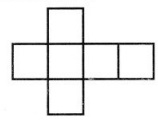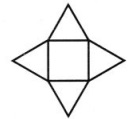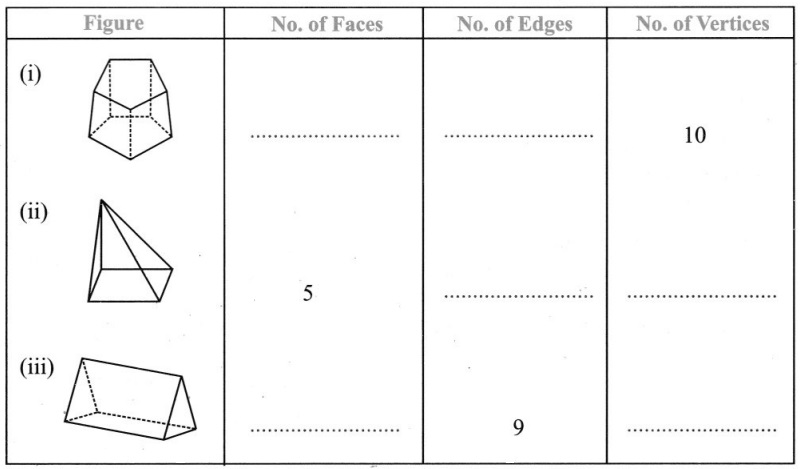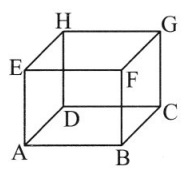# Grade 7 Visualising Solid Shapes Worksheets

## Grade 7 Maths Visualising Solid Shapes Multiple Choice Questions (MCQs)

1. Two cubes of dimensions 2 cm × 2 cm × 2 cm are placed side by side, the length of resulting cuboid is
(a) 2 cm
(b) 3 cm
(c) 4 cm
(d) 6 cm

2. Which one is a 3D shape?
(a) Rectangle
(b) circle
(c) cube
(d) square

3. A cuboid has …………….. vertices.
(a) 4
(b) 6
(c) 8
(d) 12

4. The number of faces of a cone is ……………. .
(a) 1
(b) 6
(c) 2
(d) 3

5. The number of vertices of a cone is …………. .
(a) 1
(b) 6
(c) 2
(d) 3

6. The number of faces of a triangular pyramid or tetrahedron is ……………… .
(a) 4
(b) 5
(c) 6
(d) none of these

7. The number of rectangular faces of a rectangular pyramid is ……………… .
(a) 1
(b) 4
(c) 2
(d) 3

8. The number of edges of a triangular pyramid is ………….. .
(a) 6
(b) 8
(c) 9
(d) 12

9. The number of faces of a triangular pyramid is ……………… .
(a) 6
(b) 8
(c) 4
(d) 5

10. A ………….. is a skeleton-outline of a solid that can be folded to make it
(a) vertices
(b) edges
(c) faces
(d) net

11. Which of the following is Euler’s Formula:
(a) F + V – E = 2
(b) F + V = E – 2
(c) F – V = E – 2
(d) F – V + E = 2

12. What will be the number of edges if there are 12 vertices and 20 faces?
(a) 32
(b) 28
(c) 30
(d) 42

13. What will be the number of faces if there are 6 vertices and 12 edges?
(a) 8
(b) 10
(c) 12
(d) 18

14. Name of the solid given below left figure.
(a) Pyramid
(b) Cone
(c) Cube
(d) Cuboid15. Name of the solid given above sided right figure.
(a) Pyramid
(b) Cone
(c) Cube
(d) Cuboid

16. Name of the solid whose net diagram is given in below left figure.
(a) Pyramid
(b) Cone
(c) Cube
(d) Cuboid17. Name of the solid whose net diagram is given in above sided right figure.
(a) Pyramid
(b) Cone
(c) Cube
(d) Cuboid

18. Two dice are placed side by side with 5 + 6, what is the total on the face opposite to the given numbers.
(a) 3
(b) 7
(c) 11
(d) 6

19. Two dice are placed side by side with 4 + 3, what is the total on the face opposite to the given numbers
(a) 3
(b) 7
(c) 11
(d) 6

20. What cross-sections do you get when you give a horizontal cut to an ice-cream cone?
(a) rectangle
(b) square
(c) circle
(d) triangle

### Grade 7 Maths Visualising Solid Shapes Fill In The Blanks

1. The solid with one circular face, one curved surface and one vertex is called ……………. .
2. Total no. of edges a cylinder are ……………… .
3. A solid with no vertex is ……………….. .
4. The common portion of two adjacent faces of a cuboid is called …………….. .
5. The number of vertices of a cube is …………….. .

### Grade 7 Maths Visualising Solid Shapes True(T) Or False(F)

1. In oblique sketch of the solid the measurements are kept proportional.
2. The measurements are of exact size in an isometric sketch.
3. While sphere is a 2-D figure, circle is a 3-D figure.
4. The circle, the square, the rectangle and the triangle are example of plane figures.
5. The number of faces of a cylinder is 3.

### Grade 7 Maths Visualising Solid Shapes Very Short Answer Type Questions1. Which edge is the intersection of faces EFGH and EFBA?2. which faces intersect at edge FB?
3. Give all the edges that are perpendicular to edge AB.
4. Give four vertices that do not all lie in one plane.